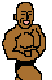## RT Cyg: Analysis of the AFOEV database

(posted by I. Andronov, vsnet 859)

\documentstyle[11pt]{article}
\textwidth 170mm
\textheight 230mm
\oddsidemargin -3mm
\topmargin -16mm
\begin{document}
\begin{center}


{\Large\bf CYCLE--TO--CYCLE CHANGES\\ IN THE MIRA--TYPE STAR RT CYG} \footnote{IAU Symposium 180 "Planetary Nebulae" Poster III-4}\\[1mm] V.I. Marsakova$^1,$ I.L. Andronov$^1,$ E. Schweitzer$^2$\\[1mm] $^1$Department of Astronomy, Odessa State University, T.G.Shevchenko Park, Odessa 270014 Ukraine\\ $^2$AFOEV, Strasbourg, 11, rue de l'Universit\'e, 67000 Strasbourg, France\\[2mm] \end{center}

{\bf Abstract}. The moments of 82 maxima and 76 minima are used for the correlation analysis of the characteristics of the individual cycles. 5 not trivial correlations were found. The characteristic time of the secular decrease of the period is $|P/\dot{P}|=(23.4\pm4.3)\cdot10^3$ yrs, but decade--scale and cycle--to--cycle variations are also present.\\[2mm]

The observations were made by the members of the AFOEV (JD 2422897 -- 50169). The AFOEV database was described by Schweitzer (1993). From all data we deleted not sure values and estimates "fainter than". We also have used our photographic data which were converted to the visual magnitudes by using the characteristic curve $m_{vis}(m_{pg}).$ For further analysis alltogether 7421 data points were used.

These data were smoothed by a multiharmonic fit by using the program by Andronov (1994). The statistically significant number of harmonics is $m=3,$ mean brightness at the maximum $7.40\pm0.02$ and minimum $11.94\pm0.02.$ The estimates of the period $P=190.162\pm.003$ and asymmetry $f=0.458\pm0.003.$

The individual cycles were fitted by the "asymptotic parabola" fit and the time and brightness of the extrema was determined with corresponding error estimates as described by Marsakova and Andronov (1996).

The moments of 82 maxima and 76 minima were fitted with weights inverse proportional to the error estimate of the moment. The initial epoch for the maximum is JD 2444589.6$\pm$0.9, for the minimum 24444499.8$\pm$0.7, the period $P=190.13\pm0.01.$ The corresponding asymmetry is $f=0.472\pm0.006.$

The value of the period has changed from $191.86\pm0.5$ days (JD 2423003--24340) to $189.4\pm0.1$ (46406--50089). Suggesting secular period change one may obtain from the moments of extrema the characteristic time of the period decrease $|P/\dot{P}|=(23.4\pm4.3)\cdot10^3$ yrs. However, the period changes are more complicated. High-order polynomial fits to the moments of extrema argue for a statistically significant value of the degree of the polynomial $n=4.$ This formal value argues for decade--scale period variations. The asymmetry estimate is practically the same as for $n=1:$ $f=0.484\pm0.005.$

For the correlation analysis we have used the brightness $m$ and time $t$ of the current (0), preceeding (1) and succeding (2) maximum, preceeding (3) and succeding (4) minimum and their combinations. The most prominent not trivial positive correlations were found for the pairs $(m_3-m_4)-(m_0-m_2),$ $(m_2-m_0)-(t_4-t_3),$ $m_0-m_3$ (or relative $m_2-m_4$,) $m_2-(m_4-m_0),$ $m_2-(m_4-m_3).$\\[2mm]

{\bf References}\\[2mm]
Andronov, I.L., 1994, Odessa Astron. Publ., 7, 49.\\
Marsakova, V.I., Andronov, I.L., 1996, Odessa Astron. Publ., 9, in press.\\
Schweitzer, E.: 1993,  Bull. AFOEV,  64, 14.
\end{document}


vsnet-adm@kusastro.kyoto-u.ac.jp